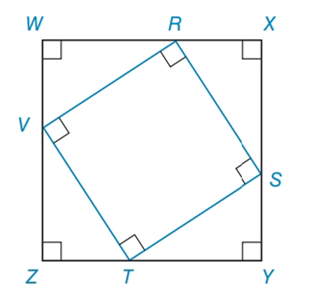Chapter 8.2, Problem 39EElementary Geometry For College St...

7th Edition
Alexander + 2 others
ISBN: 9781337614085

Solutions

Chapter
SectionElementary Geometry For College St...

7th Edition
Alexander + 2 others
ISBN: 9781337614085
Textbook Problem

Square R S T V is inscribed in square W X Y Z , as shown. If Z T = 5 and T Y = 12 , finda) the perimeter of R S T V b) the area of R S T VExercises 39, 40

To determine

(a)

To find:

The perimeter of the square.

Explanation

Formula Used:

The perimeter of a polygon is the sum of the lengths of all sides of the polygon.

For quadrilateral, P=2(b+h), where b is the base and h is the altitude of the quadrilateral.

Calculation:

It is given that the square RSTV is inscribed in square WXYZ with ZT=5 and TY=12.

The side ZY is split into ZT and TY with dimension 5 and 12 respectively.

As WXYZ is a square, each side of RSTV can also be split into two parts. That is, 5 and 12 respectively. Therefore, ZT=YS=XR=WV=5 and TY=SX=RW=VZ=12

To determine

(b)

To find:

The area of the square.

Still sussing out bartleby?

Check out a sample textbook solution.

See a sample solution

The Solution to Your Study Problems

Bartleby provides explanations to thousands of textbook problems written by our experts, many with advanced degrees!

Get Started

In Exercises 516, evaluate the given quantity. log1616

Finite Mathematics and Applied Calculus (MindTap Course List)

Simplify: 1218

Elementary Technical Mathematics

In Problems 13-26, graph the solution of each system of inequalities. 26.

Mathematical Applications for the Management, Life, and Social Sciences

Evaluate the integral. 50. 1x24x+1dx

Single Variable Calculus: Early Transcendentals

(y)2 + 6xy 2y + 7x = 0 is a differential equation of order ______. a) 1 b) 2 c) 3 d) 4

Study Guide for Stewart's Single Variable Calculus: Early Transcendentals, 8th

For and , a × b =

Study Guide for Stewart's Multivariable Calculus, 8th